Next: The electric scalar potential Up: Time-independent Maxwell equations Previous: Introduction

## Coulomb's law

Between 1785 and 1787, the French physicist Charles Augustine de Coulomb performed a series of experiments involving electric charges, and eventually established what is nowadays known as Coulomb's law. According to this law, the force acting between two electric charges is radial, inverse-square, and proportional to the product of the charges. Two like charges repel one another, whereas two unlike charges attract. Suppose that two charges,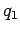and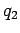, are located at position vectors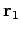and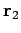. The electrical force acting on the second charge is written(161)

in vector notation (see Fig. 24). An equal and opposite force acts on the first charge, in accordance with Newton's third law of motion. The SI unit of electric charge is the coulomb (C). The magnitude of the charge on an electron is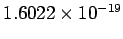C. The universal constantis called the permittivity of free space, and takes the value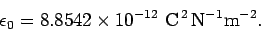(162)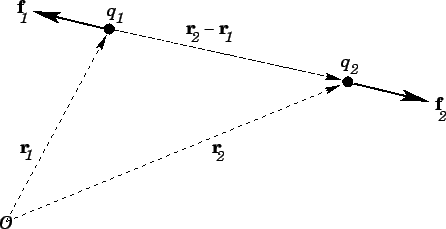Coulomb's law has the same mathematical form as Newton's law of gravity. Suppose that two masses,and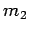, are located at position vectorsand. The gravitational force acting on the second mass is written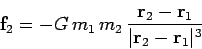(163)

in vector notation. The gravitational constant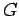takes the value(164)

Coulomb's law and Newton's law are both inverse-square force laws: i.e.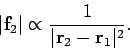(165)

However, they differ in two crucial respects. Firstly, the force due to gravity is always attractive (there is no such thing as a negative mass). Secondly, the magnitudes of the two forces are vastly different. Consider the ratio of the electrical and gravitational forces acting on two particles. This ratio is a constant, independent of the relative positions of the particles, and is given by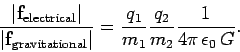(166)

For electrons, the charge to mass ratio is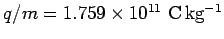, so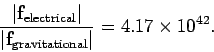(167)

This is a colossal number! Suppose we are studying a physics problem involving the motion of particles in a box under the action of two forces with the same range, but differing in magnitude by a factor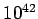. It would seem a plausible approximation (to say the least) to start the investgation by neglecting the weaker force. Applying this reasoning to the motion of particles in the Universe, we would expect the Universe to be governed entirely by electrical forces. However, this is not the case. The force which holds us to the surface of the Earth, and prevents us from floating off into space, is gravity. The force which causes the Earth to orbit the Sun is also gravity. In fact, on astronomical length-scales gravity is the dominant force, and electrical forces are largely irrelevant. The key to understanding this paradox is that there are both positive and negative electric charges, whereas there are only positive gravitational charges.'' This means that gravitational forces are always cumulative, whereas electrical forces can cancel one another out. Suppose, for the sake of argument, that the Universe starts out with randomly distributed electric charges. Initially, we expect electrical forces to completely dominate gravity. These forces try to make every positive charge get as far away as possible from the other positive charges, and as close as possible to the other negative charges. After a while, we expect the positive and negative charges to form close pairs. Just how close is determined by quantum mechanics, but, in general, it is pretty close: i.e., about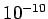m. The electrical forces due to the charges in each pair effectively cancel one another out on length-scales much larger than the mutual spacing of the pair. It is only possible for gravity to be the dominant long-range force if the number of positive charges in the Universe is almost equal to the number of negative charges. In this situation, every positive change can find a negative charge to team up with, and there are virtually no charges left over. In order for the cancellation of long-range electrical forces to be effective, the relative difference in the number of positive and negative charges in the Universe must be incredibly small. In fact, positive and negative charges have to cancel each other out to such accuracy that most physicists believe that the net charge of the universe is exactly zero. But, it is not enough for the Universe to start out with zero charge. Suppose there were some elementary particle process which did not conserve electric charge. Even if this were to go on at a very low rate, it would not take long before the fine balance between positive and negative charges in the Universe was wrecked. So, it is important that electric charge is a conserved quantity (i.e., the net charge of the Universe can neither increase or decrease). As far as we know, this is the case. To date, no elementary particle reactions have been discovered which create or destroy net electric charge.

In summary, there are two long-range forces in the Universe, electromagnetism and gravity. The former is enormously stronger than the latter, but is usually hidden'' away inside neutral atoms. The fine balance of forces due to negative and positive electric charges starts to break down on atomic scales. In fact, interatomic and intermolecular forces are all electrical in nature. So, electrical forces are basically what prevent us from falling though the floor. But, this is electromagnetism on the microscopic or atomic scale--what is usually termed quantum electromagnetism. This course is about classical electromagnetism. That is, electromagnetism on length-scales much larger than the atomic scale. Classical electromagnetism generally describes phenomena in which some sort of violence'' is done to matter, so that the close pairing of negative and positive charges is disrupted. This allows electrical forces to manifest themselves on macroscopic length-scales. Of course, very little disruption is necessary before gigantic forces are generated. It is no coincidence that the vast majority of useful machines which humankind has devised during the last century or so are electrical in nature.

Coulomb's law and Newton's law are both examples of what are usually referred to as action at a distance theories. According to Eqs. (161) and (163), if the first charge or mass is moved then the force acting on the second charge or mass immediately responds. In particular, equal and opposite forces act on the two charges or masses at all times. However, this cannot be correct according to Einstein's theory of relativity, which implies that the maximum speed with which information can propagate through the Universe is the speed of light in vacuum. So, if the first charge or mass is moved then there must always be time delay (i.e., at least the time needed for a light signal to propagate between the two charges or masses) before the second charge or mass responds. Consider a rather extreme example. Suppose the first charge or mass is suddenly annihilated. The second charge or mass only finds out about this some time later. During this time interval, the second charge or mass experiences an electrical or gravitational force which is as if the first charge or mass were still there. So, during this period, there is an action but no reaction, which violates Newton's third law of motion. It is clear that action at a distance is not compatible with relativity, and, consequently, that Newton's third law of motion is not strictly true. Of course, Newton's third law is intimately tied up with the conservation of linear momentum in the Universe. This is a concept which most physicists are loath to abandon. It turns out that we can rescue'' momentum conservation by abandoning action at a distance theories, and instead adopting so-called field theories in which there is a medium, called a field, which transmits the force from one particle to another. In electromagnetism there are, in fact, two fields--the electric field, and the magnetic field. Electromagnetic forces are transmitted via these fields at the speed of light, which implies that the laws of relativity are never violated. Moreover, the fields can soak up energy and momentum. This means that even when the actions and reactions acting on particles are not quite equal and opposite, momentum is still conserved. We can bypass some of the problematic aspects of action at a distance by only considering steady-state situations. For the moment, this is how we shall proceed.

Considercharges,though, which are located at position vectorsthrough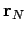. Electrical forces obey what is known as the principle of superposition. The electrical force acting on a test charge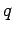at position vectoris simply the vector sum of all of the Coulomb law forces from each of thecharges taken in isolation. In other words, the electrical force exerted by the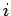th charge (say) on the test charge is the same as if all the other charges were not there. Thus, the force acting on the test charge is given by(168)

It is helpful to define a vector field, called the electric field, which is the force exerted on a unit test charge located at position vector. So, the force on a test charge is written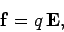(169)

and the electric field is given by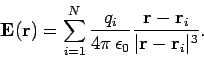(170)

At this point, we have no reason to believe that the electric field has any real physical existence. It is just a useful device for calculating the force which acts on test charges placed at various locations.

The electric field from a single chargelocated at the origin is purely radial, points outwards if the charge is positive, inwards if it is negative, and has magnitude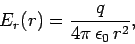(171)

where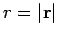.We can represent an electric field by field-lines. The direction of the lines indicates the direction of the local electric field, and the density of the lines perpendicular to this direction is proportional to the magnitude of the local electric field. Thus, the field of a point positive charge is represented by a group of equally spaced straight lines radiating from the charge (see Fig. 25).

The electric field from a collection of charges is simply the vector sum of the fields from each of the charges taken in isolation. In other words, electric fields are completely superposable. Suppose that, instead of having discrete charges, we have a continuous distribution of charge represented by a charge density. Thus, the charge at position vectoris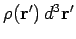, where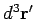is the volume element at. It follows from a simple extension of Eq. (170) that the electric field generated by this charge distribution is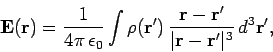(172)

where the volume integral is over all space, or, at least, over all space for which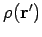is non-zero.Next: The electric scalar potential Up: Time-independent Maxwell equations Previous: Introduction
Richard Fitzpatrick 2006-02-02